Ch 7. Stress Analysis Multimedia Engineering Mechanics PlaneStress PrincipalStresses Mohr's Circlefor Stress Failure PressureVessels
 Chapter 1. Stress/Strain 2. Torsion 3. Beam Shr/Moment 4. Beam Stresses 5. Beam Deflections 6. Beam-Advanced 7. Stress Analysis 8. Strain Analysis 9. Columns Appendix Basic Math Units Basic Equations Sections Material Properties Structural Shapes Beam Equations Search eBooks Dynamics Fluids Math Mechanics Statics Thermodynamics Author(s): Kurt Gramoll ©Kurt GramollMECHANICS - THEORY

Stress Rotation Equation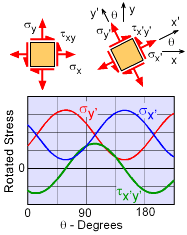Rotated Stresses using
Stress Rotation Equations

Previously, the stress transformation equations were developed to calculate the stress state at different orientations. These equations were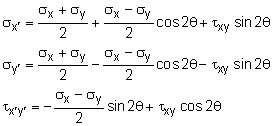Plotting these equations show that every 180 degrees rotation, the stress state repeats. In 1882, Otto Mohr noticed that these relationships could be graphically represented with a circle. This was a tremendous help in the days of slide rulers when using complex equations, like the stress transformation equations, was time consuming.

Mohr's Circle

Mohr's circle is not actually a new derived formula, but just a new way to visualize the relationships between normal stresses and shear stresses as the rotation angle changes. To determine the actual equation for Mohr's circle, the stress transformation equations can be rearranged to give,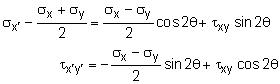Each side of these equations can be squared and then added together to give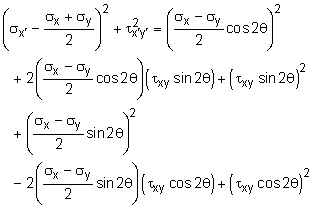Grouping similar terms and canceling other terms gives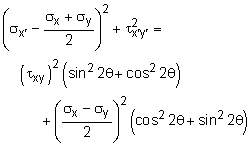Using the trigonometry identity, cos22θ + sin22θ = 1, gives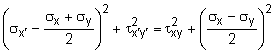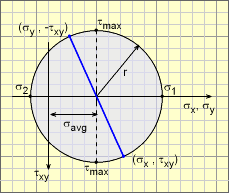Basic Mohr's Circle for Stress

This is basically an equation of a circle. The circle equation can be better visualized if it is simplified to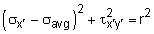where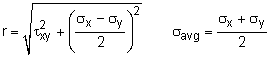This circle equation is plotted at the left using r and σave. One advantage of Mohr's circle is that the principal stresses, σ1, σ2 , and the maximum shear stress, τmax, are easily identified on the circle without further calculations.

Rotating Stresses with Mohr's Circle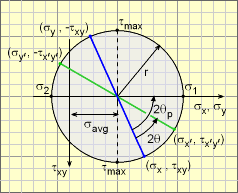Stress Rotation with Mohr's Circle

In addition to identifying principal stress and maximum shear stress, Mohr's circle can be used to graphically rotate the stress state. This involves a number of steps.

1. On the horizontal axis, plot the circle center at σavg = (σx + σy)/2.
2. Plot either the point (σx , τxy) or (σy , -τxy). Note the sign change if plotting σy and the vertical axis, τxy, is positive downward.
3. Draw a line from the center to the point plotted in step two (blue line in the diagram). This line should extend from one side of the circle to the other. Radius, r, can now be measured from the graph.
4. The circle itself can be drawn since the center and one point on the circle is known (compass works well for this).
5. The principal stresses and maximum shear stress can be identified on the graph.
6. The line drawn in step 3 can be rotated twice the rotation angle, 2θ, in the counter-clockwise direction. It is important that the angle is twice the desired rotated angle.
7. The new stress state is the intersection of the new line (green in the diagram) and the circle.

The angle, 2θp, for the principal stresses is simply the half the angle from the blue line to the horizontal axis.

Remember, Mohr's circle is just another way to visualize the stress state. It does not give additional information. Both the stress transformation equations and Mohr's circle will give the exactly same values.

Practice Homework and Test problems now available in the 'Eng Mechanics' mobile app
Includes over 400 problems with complete detailed solutions.
Available now at the Google Play Store and Apple App Store.